# Calculated column for calculated total

Hello,

I have added 2 calculations that reflects the min value of my m1.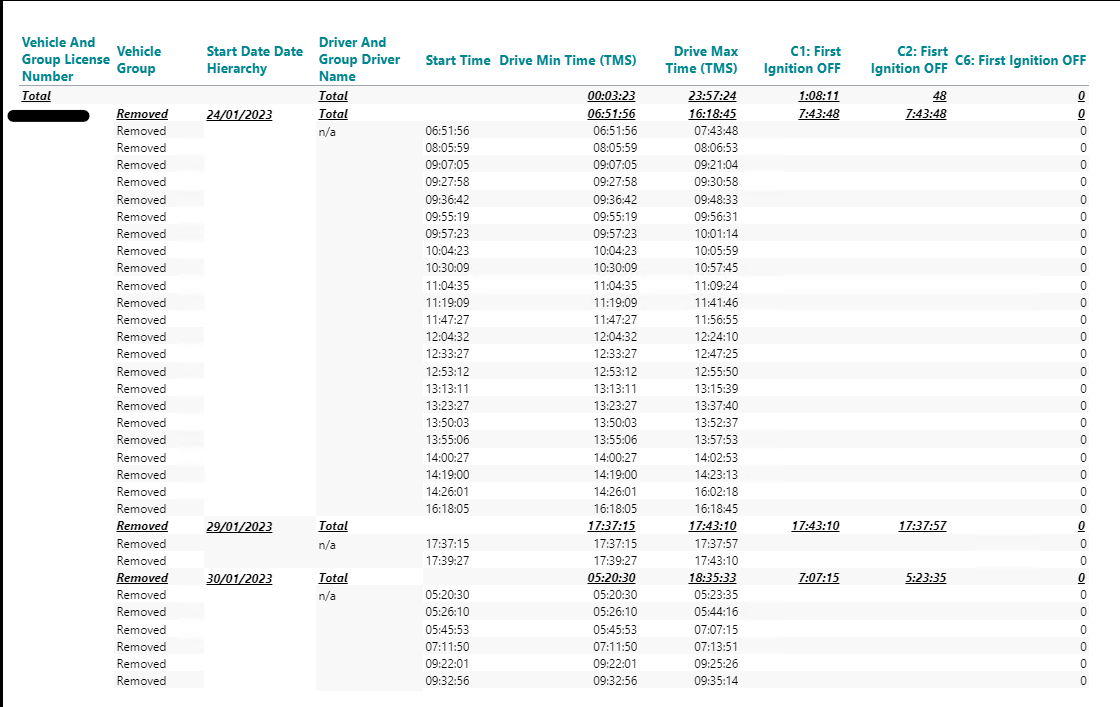Now I'm struggling to get the calculated total min value in a column, so that I can use it for an additional calculation using a statement if x then x else x (C2_ First Ignition OFF) to be able to apply a visibility agent at the end.

The calculation returning 0 (C6) is following - if allcount(d1, d1:0(l(1,0,0,0,0)), m1) > 1 then sum(c2, 0(l(1,0,0)), m1) else 0 (multiple vehicle dimension members are being present). There are 5 dimensions and 2 measures.

Does anyone have a suggestion on how this could be solved?

I also need to have additional columns for my m2 using the MAX syntax.

Br.

Steffi

• Hi Steffi,

I need more information in order to understand the issue here. Can you modify the screenshot, e.g., export to Excel and insert the expected values in the right places. That will make it easier for me to understand what it is you want to achieve.

BR / Ole

• Hi Ole,

please see below. I have filled in the data in column J based on the calculations that are already part of my report. I have also added column K:M in order to show what exactly I want to achieve.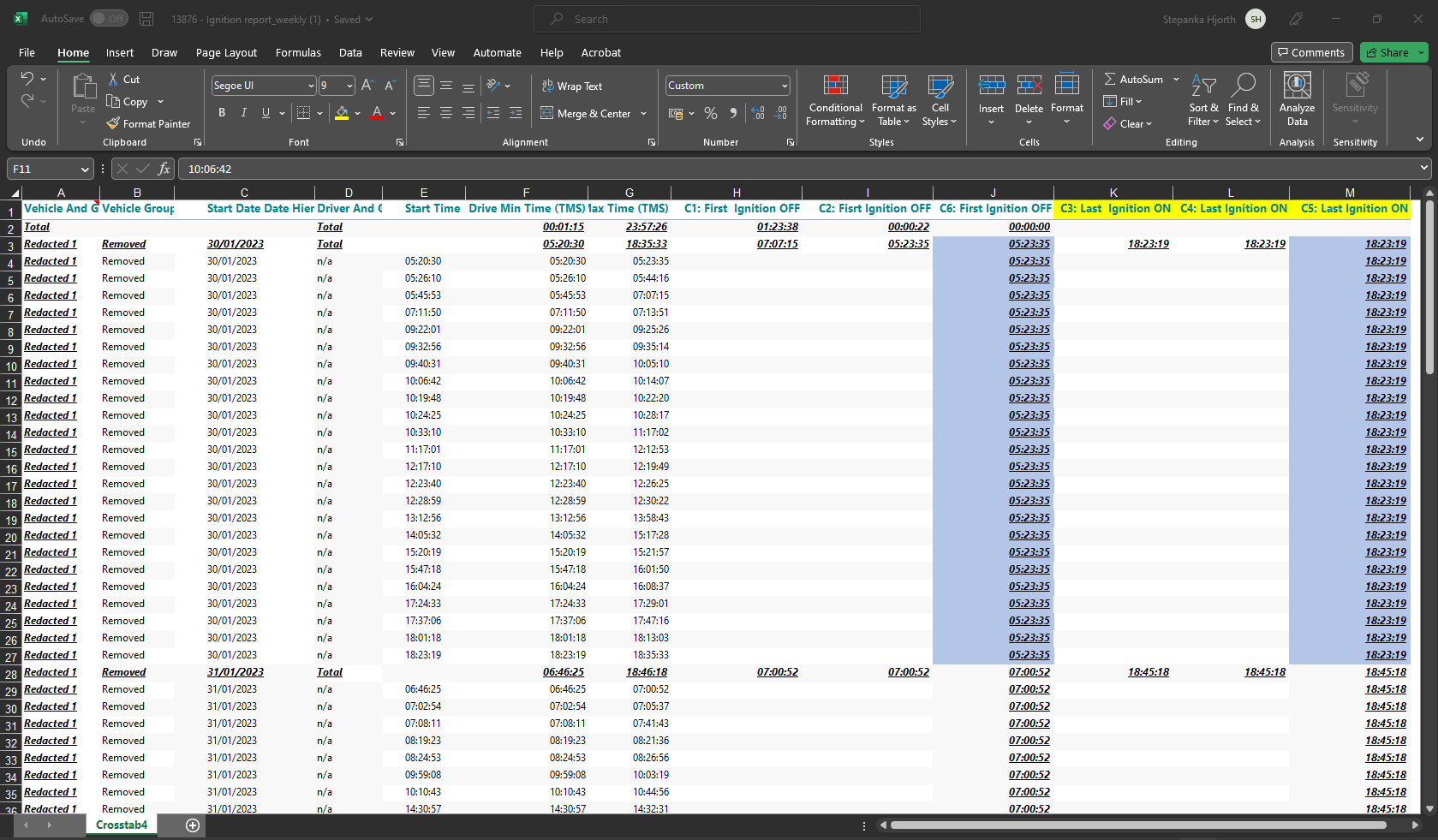I need to calculate the MIN value from column G and the MAX value from column F per dimension member and date. Once I have the right values for the first and last ignition status, I'll add 2 new calculations if x then 1 else 0 to be able to hide members that are irrelevant for the report.

Thank you!

/Steffi

• Hi Steffi,

I am still not sure if you just need the MIN and MAX for further calculations - or if you also need the subtotals to display something meaningful...

Also, I am interpreting 'MIN value from column G' as first value of m2 (within a given date) and 'MAX value from column F' as last value of m1 (within a given date).

In my example, I am using the following two calculations:

`First m2: sum(d1, d1(s), m2)Last m1: sum(d1, d-1(s), m1)`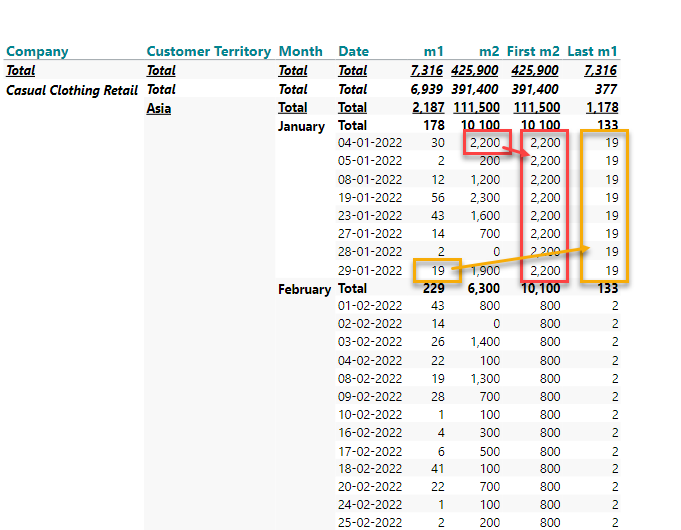Let me know if I am on the right path?

BR / Ole

• Hi Ole,

unfortunately not. I'm trying to get the calculated total from my time measures in a column.

Maybe this screenshot illustrates it better?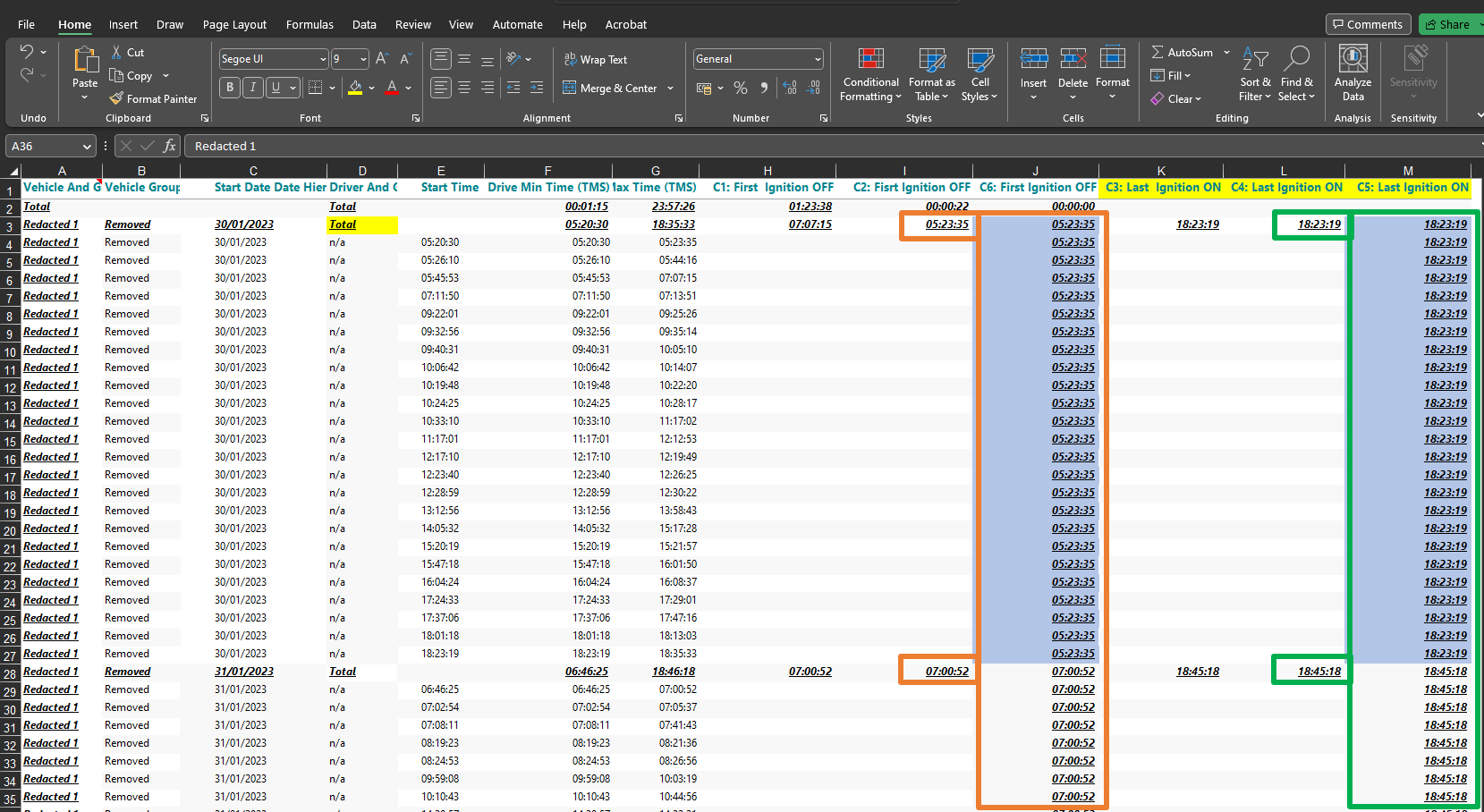I have tried to work with siblings to start with but that just sum's the values as they are and does not return the MIN/MAX of my time measures.

/Steffi

• Hi Steffi,

I am sorry, but I am no less confused.

What are the calculatiosn behind C2 and C4 (and C1 and C3)?

BR / Ole

• Hi Ole,

ok, here some more details then. These are the dimensions and measures used.: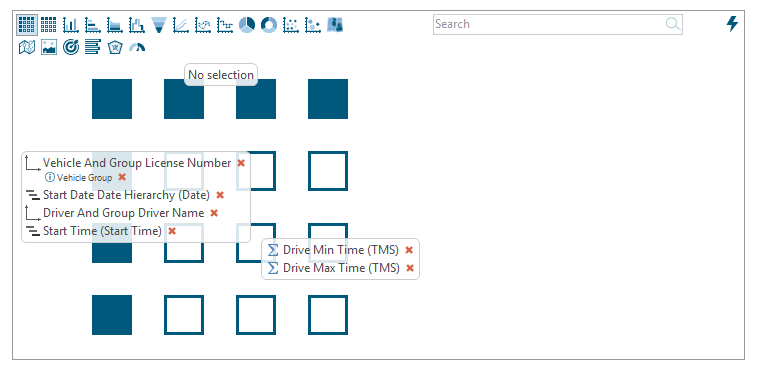As the first calculation did not always return the correct minimum value, I have added the same calculation as the first one just based on the newly calculated values.: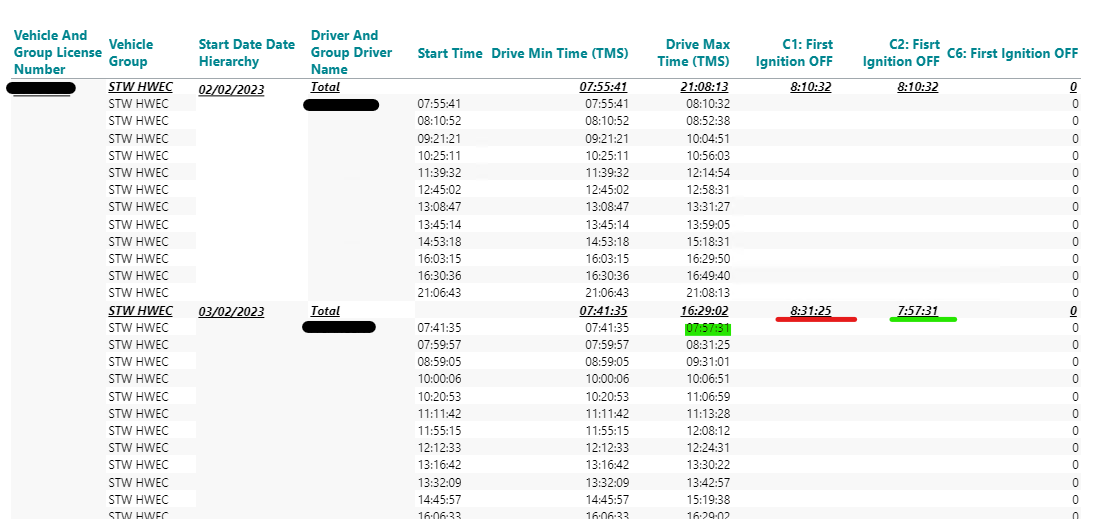C1: First  Ignition OFF =  min(d1, all(c), m2) (m2 is Drive MAX time TMS)

C2: First Ignition OFF = min(c1, all(c), m1) (c1 is C1: First  Ignition OFF)

For the example above should C6: First Ignition OFF return the value 07:57:31 instead of a zero.

For C3 and C4 it is more or less the same logic based on m1.: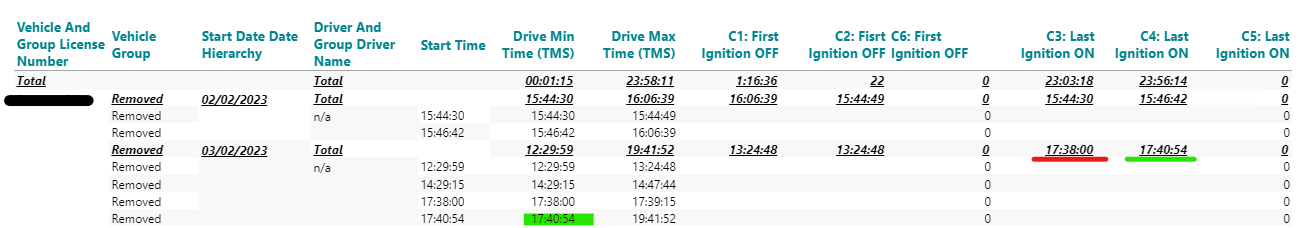C3: Last  Ignition ON = max(d1, all(c), m1) (m1 is Drive MIN Time TMS)

C4: Last  Ignition ON = max(c4, all(c), m1) (c4 is C3: Last  Ignition ON)

For the example above C5: Last Ignition ON should return 17:40:54 instead of an zero.

Does this make more sense now?

Thank you

/Steffi

• Please, can you also provide a screenshot of your visibility settings. I wonder if some of the subtotals have been hidden?

• you are correct, some data sets has been "hidden".: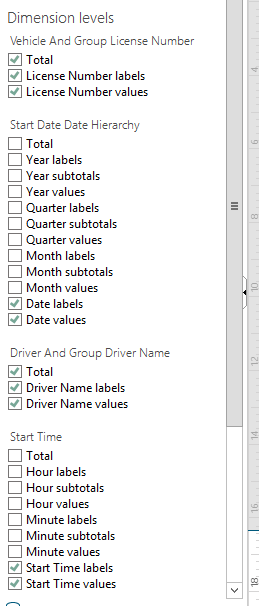• All right,

It is very hard to understand - and to replicate - when I don't have all the details... :-)

You have four dimensions on the vertical axis (the first two columns, 'Vehicle And Group License Number' and 'Vehicle Group' are treated as one dimension, with a member property).

With four dimensions, you can create level-specific calculations such as

`sum(c2, 0(l(1,0,0,0)), m1)`

or

`sum(c2, 0(l(1,1,0,0)), m1)`

or

`sum(c2, 0(l(1,1,1,0)), m1)`

Please try one of the above as your C6 calculation until you get the result from the right level in your crosstab.

BR / Ole

• I understand :-) and it's not always easy to provide all required information's at first.

I have tested the calculation's you have provided and I believe we are getting closer.

Now I'm getting values in but its the MIN (C6 First Ignition OFF) on vehicle level and not on date level.

The one with the green arrow would be the correct one for that date.• Forgot to mention that I'm using sum(c2, 0(l(1,1,0,0)), m1) in C6.

/Steffi

• Hi Ole,

did the above provide a more detailed explanation?

I btw use five dimensions and not four as you mentioned previously. Is that maybe the key for a solution?

Thank you.

/Steffi

• Yes. The number of dimensions does make a difference to the formula.

Example, for four dimensions: sum(c2, 0(l(1,1,0,0)), m1)

Example, for five dimensions: sum(c2, 0(l(1,1,0,0,0)), m1)

The number of parameters (1s or 0s) inside the brackets must match the number of dimensions.

BR / Ole

• Thank you, Ole. This is what I thought and I have tested myself the sum(c2, 0(l(1,1,0,0,0)), m1), however it still returns the values per license number dimension, rather then the MIN (lowest time stamp) per day.

How can I change the calculation to look up the MIN per date and not license number? Or is that not possible?

Many thanks

/Steffi

• Have you tried with sum(c2, 0(l(1,0,0,0,0)), m1)?

Or with sum(c2, 0(l(1,1,1,0,0)), m1)?

Or with sum(c2, 0(l(1,1,1,1,0)), m1)?

Or with sum(c2, 0(l(1,1,1,1,1)), m1)?

Again, it is not easy for me to tell the exact formula, when I don't have the data.

BR / Ole

• Yes, I have tried all of these and the last 3 returns undefined.

Should I open a JIRA ticket instead?

/Steffi